# DAV Class 5 Maths Chapter 2 Brain Teasers Solutions

The DAV Class 5 Maths Book Solutions Pdf and DAV Class 5 Maths Chapter 2 Brain Teasers Solutions of Operations on Large Numbers offer comprehensive answers to textbook questions.

## DAV Class 5 Maths Ch 2 Brain Teasers Solutions

Question 1.
(a) The sum of the greatest 4-digit number and the smallest 6-digit number is ______
(i) 1099999
(ii) 109999
(iii) 900000
(iv) 199999
Solution:
Greatest 4-digit number = 9999
Smallest 6-digit number = +100000
Sum = 109999
So, option (ii) 1,09,999 is correct.

(b) 9999 ÷ 99 = ______
(i) 11
(ii) 101
(iii) 99
(iv) 110
Solution: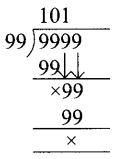So, option (ii) 101 is correct.

(c) Digit in the one’s place of the product of 783 and 896 is ________
(i) 9
(ii) 2
(iii) 8
(iv) 6
Solution:
Product of one place of 783 and 896
= 3 × 6
= 18
So, option (iii) is correct.(d) Digit in the hundreds place of the difference of 53883 and 41834 is __________
(i) 9
(ii) 2
(iii) 0
(iv) 4
Solution:
53883 – 41834 = 12049
So, option (iii) is correct.

(e) Tens digit in the sum of 13846, 62381, 57402 is __________
(i) 2
(ii) 3
(iii) 4
(iv) 5
Solution:
13846 + 62381 + 57402 = 133629
So, option (i) is correct.

Question 2.
Replaceby the correct digit.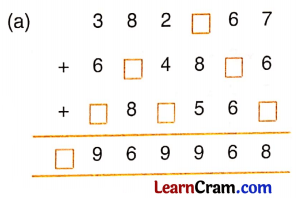Solution: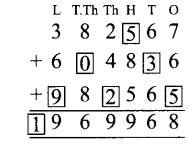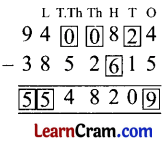Question 3.
Find the product.
(a) 9,80,406 × 708
Solution:(b) 5,67,894 × 625
Solution:Question 4.
Divide
(a) 99,99,999 ÷ 9,999
Solution: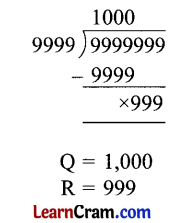(b) 6,85,432 ÷ 234
Solution: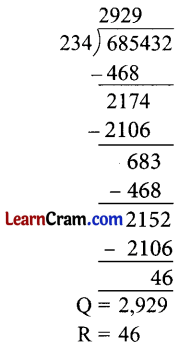Question 5.
Subtract 93,84,236 from the sum of 3,95,08,625 and 74,38,906.
Solution:Question 6.
The total number of men, women, and children in a state is 93,86,493. If the number of men is 26,38,755 and that of women is 25,29,431, find the number of children.
Solution:
To find the number of children we have to subtract the total population from no. of men & no. of women.
No. of men = 2638755
No. of women = +2529431
No. of men and women = 5168186
Total no. of population = 9386493
No. of men and women = -5168186
No. of children = 4218307
Number of children is 42,18,307.

Question 7.
A dealer purchased 285 washing machines. If the cost of one washing machine is ₹ 9,825, find the cost of the purchased washing machines.
Solution:
Cost of 1 machine= ₹ 9,825
Costof285 machines = ₹ 9825 × 285 = ₹ 28,00,125
The cost of the purchased washing machines is ₹ 28,00,125.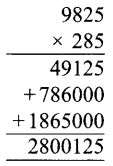Question 8.
Find the product of the greatest 5-digit and 3-digit numbers.
Solution:
Greatest 5-digit number = 99999
Greatest 3-digit number = 999
Their Product = 99999 × 999 = 99899001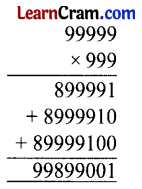Question 9.
An engine pumps 2,85,000 litres of water in five hours. How many litres of water will the engine pump in one minute?
Solution:
1 hour = 60 minutes
5 hour = 300 minutes
In 300 minutes engine pumps water = 285000 litres.
In 1 minute engine pumps water = 285000 ÷ 300 = 950 litres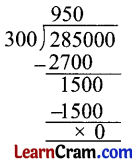Question 10.
Find the value of 5,43,86,291 + 1,09,853 – 96,298.
Solution: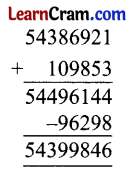Question 1.
(a) Digit in one place of the product of 189 and 179 is
(i) 1
(ii) 9
(iii) 3
(iv) 6
Solution:
(i) 1

(b) The sum of the greatest 5-digit number and the smallest 6-digit number is
(i) 10999
(ii) 199999
(iii) 198699
(iv) 199969
Solution:
(ii) 199999

(c) Digit in the tens place of the difference of 68719 and 32160 is
(i) 4
(ii) 5
(iii) 6
(iv) 7
Solution:
(ii) 5(d) Digit in the hundreds place of the sum of 13502, 78961, and 57302 is
(i) 5
(ii) 6
(iii) 1
(iv) 8
Solution:
(iii) 1

Question 2.
(a) 50,71,993; 23,73,473; 38,21,344
Solution: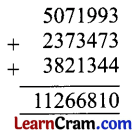(b) 5,32,373; 17,00,299; 56,37,133
Solution:(c) 5,46,113; 3,43,23,220; 9,43,56,117
Solution: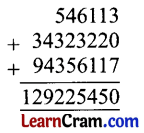Question 3.
Subtract.
(a) 4,89,94,290 from 9,70,30,490
Solution:(b) 37,97,789 from 5,26,87,870
Solution:(c) 58,70,130 from 2,70,30,390
Solution: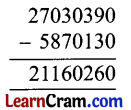Question 4.
Multiply large numbers.
(a) 26,330 × 490
Solution:(b) 10,410 × 385
Solution:(c) 7931 × 4132
Solution: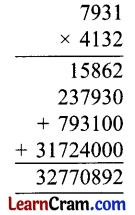Question 5.
(a) 59,70,280 ÷ 273
Solution: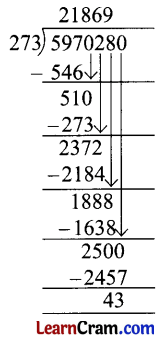Check:
Dividend = Divisor × Quotient + Remainder
R.H.S = 273 × 21869 + 43
= 5970237 + 43
= 5970280
= L.H.S(b) 56,970 ÷ 180
Solution: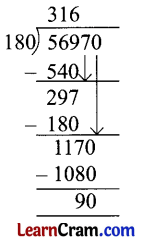Check:
Dividend = Divisor × Quotient + Remainder
R.H.S =180 × 316 + 90
= 56880 + 90
= 56970
= R.H.S

(c) 15,40,130 ÷ 75
Solution:Check:
Dividend = Divisor × Quotient + Remainder
R.H.S = 75 × 20535 + 5
= 1540125 + 5
= L.H.S.

(d) 9,00,763 ÷ 27
Solution: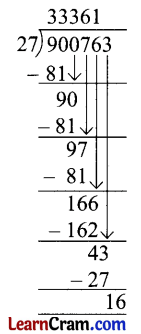Check:
Dividend = Divisor × Quotient + Remainder
R.H.S = 27 × 33361 + 16
= 900747 + 16
= 900763
= L.H.S

Question 6.
A man earns ₹ 80,350 every month. He spent ₹ 50,450 every month. What is his saving in 2 months.
Solution:
Total earning = ₹ 80350
Spent = – ₹ 50450
Saving = 29900
Saving in 1 month = ₹ 29,900
Saving in 2 months = 2 × ₹ 29900 = ₹ 59800
His saving is ₹ 59800 in 2 months.Question 7.
A dealer purchased 180 A.C. If the cost of one A.C. is ₹ 35,780, find the cost of purchased A.C.’s.
Solution:
A dealer purchased 180 A.C.
Cost of one A.C. = ₹ 35,780
Cost of 180 A.C.’s = 180 × ₹ 35,780 = ₹ 64,40,400